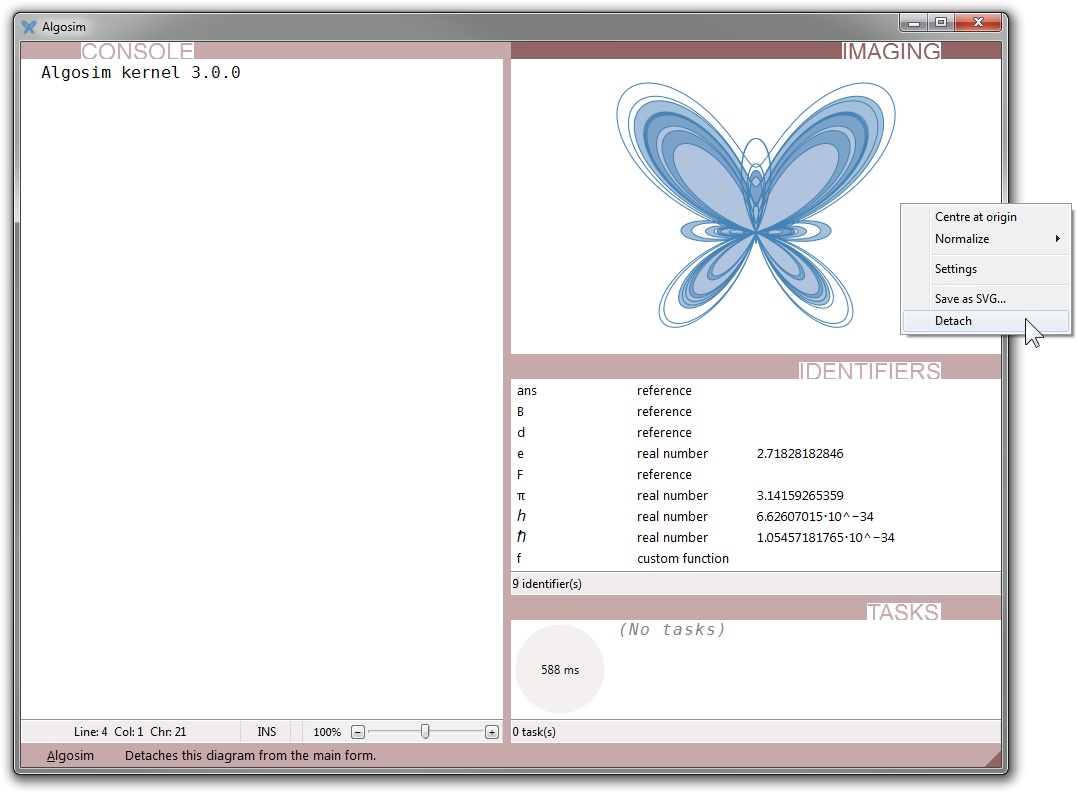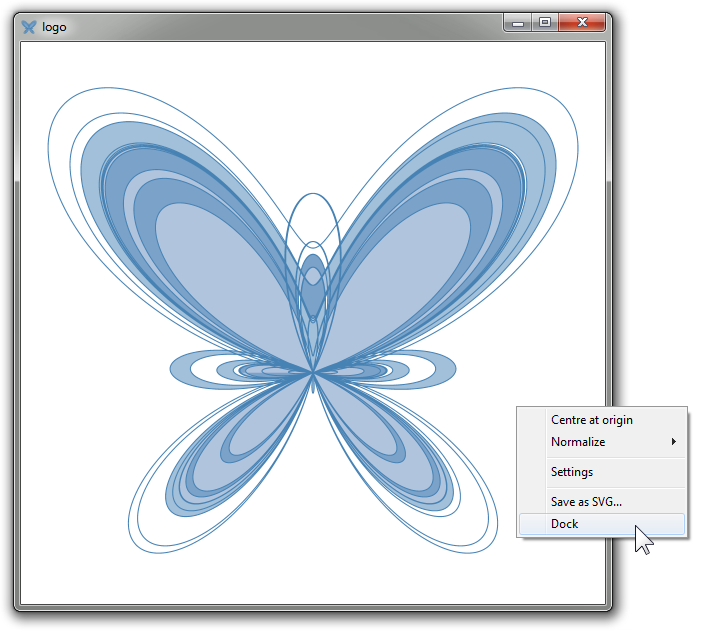﻿ diagram – Algosim documentation
Algosim documentation: diagram

# diagram

Sets the current diagram.

## Syntax

• `diagram()`

• `diagram(S)`

• `S` is a non-empty string

## Description

`diagram(S)` sets the current diagram to the one named `S`; if there is no diagram named `S`, a new diagram with this name is created.

All subsequent chart drawing and plotting operations will take place in this diagram.

A reference to the diagram is returned.

`diagram()` instructs the front-end to put each new chart or plot in its own, newly created, and temporary diagram. As soon as a new diagram (named or temporary) is displayed, the old temporary diagram is discarded, along with all objects it contains.

A named diagram can be displayed either docked in the main IDE window or floating in its own window. By right-clicking the diagram, you can detach a docked diagram or dock a detached diagram: